Refer to our Texas Go Math Grade 3 Answer Key Pdf to score good marks in the exams. Test yourself by practicing the problems from Texas Go Math Grade 3 Lesson 14.1 Answer Key Addition Strategies.

Unlock the Problem

The table shows how many musicians are in each section of a symphony orchestra. How many musicians play either string or woodwind instruments?One Way Use the number line to find 57 + 15.

A. Count on to the nearest ten. Then count by tens and ones.B. Count by tens. Then count by ones.Complete the equation
57 + 15 = ____________
So, __________ musicians play either string or woodwind instruments.

Remember
An equation is a number sentence that uses the equal sign to show that two amounts are equal.

57 + 15 = 72
So, 72 musicians play either string or woodwind instruments.

Try This! Find 43 + 28. Draw jumps and label the number line to show your thinking. Then complete the equation.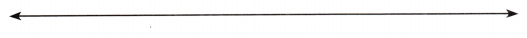So, 43 + 28 = __________.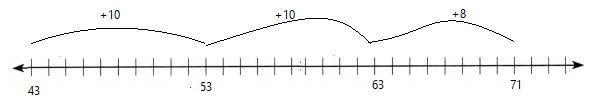So, 43 + 28 = 71

Another Way Use strip diagrams to solve an addition problem.

During a fundraiser, the band sold 132 candles in September, 161 candles in October, and 125 candles in November. How many candles did the band sell in all?

STEP 1:
Complete the strip diagram to find the number of candles sold in September and October.Then complete the equations.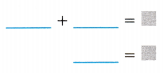STEP 2:
Complete the strip diagram to find the number of candles sold in all.
Then complete the equations.So, the band sold ________ candles in all.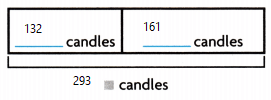Then complete the equations.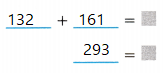STEP 2:
Complete the strip diagram to find the number of candles sold in all.
Then complete the equations.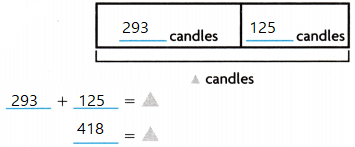So, the band sold 418 candles in all.

Share and Show

Question 1.
Count by tens and ones to find 63 + 27. Draw jumps and label the number line to show your thinking. Then complete the equation.
Think: Count by tens and ones from 63.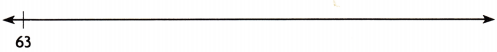63 + 27 = ___________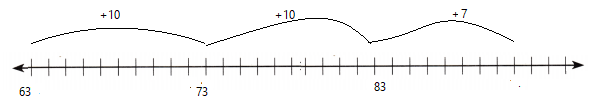Math Talk
Mathematical Processes

Explain another way you draw the jumps.Explanation:
Count on to the nearest ten. Then count by tens and ones.
63 + 27 = 90

Complete the strip diagram to represent and solve. Then complete the equation for the strip diagram.

Question 2.
There are 512 students at Fairview Elementary. There are 454 students at Lincoln Elementary. How many students attend the two schools?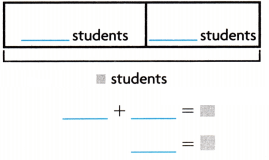Explanation:
966 students attend the two schools.

Problem Solving

Use the table for 3-6. You may use models, number lines, and equations to represent and solve.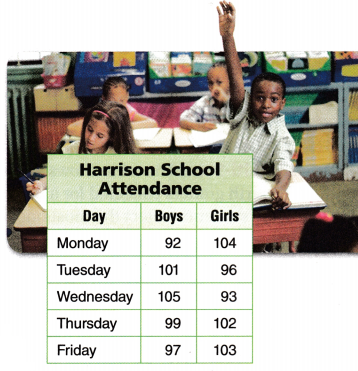Question 3.
Apply How many boys attended school on Thursday and Friday?
Explanation:
99 boys + 97 boys  = 196
196 boys attended school on Thursday and Friday

Question 4.
Analyze How many girls attended school on Monday and Tuesday?
Explanation:
104 + 96 = 200
200 girls attended school on Monday and Tuesday

Question 5.
H.O.T. Multi-Step How many students attended school on Tuesday and Wednesday? Explain how you found your answer.Explanation:
101 boys + 96 girls = 197 Tuesday
105 boys + 93 girls = 198 Wednesday
197 + 198 = 395 students attended school on Tuesday and Wednesday.

Question 6.
H.O.T. What’s the Question The answer is 201 students.
Answer: How many students attended school on Thursday?
Explanation:
99 boys + 102 girls = 201 Students.

Fill in the bubble for the correct answer choice. You can use models, number lines, and equations to represent and solve.

Question 7.
Use Tools The Juice Hut sold 27 blueberry smoothies on Monday and 25 blueberry smoothies on Tuesday. How many blueberry smoothies were sold in all on Monday and Tuesday?
(A) 47
(B) 52
(C) 60
(D) 50
Explanation:
The Juice Hut sold 27 blueberry smoothies on Monday and 25 blueberry smoothies on Tuesday
27 + 25 = 52 blueberry smoothies were sold in all on Monday and Tuesday

Question 8.
There are 198 adults and 124 children visiting a museum. What is the total number of people visiting the museum?
(A) 432
(B) 302
(C) 322
(D) 424
Explanation:
There are 198 adults and 124 children visiting a museum.
198 + 124 = 322
322 is the total number of people visiting the museum.

Question 9.
Multi-Step The table shows the number of books third and fourth graders collected for a book drive. In which week did the students collect the most books?(A) Week 4
(B) Week 2
(C) Week 3
(D) Week 1
Explanation:
Books collected in Week 1
24 + 31 = 55
Books collected in Week 2
38 + 21 = 59
Books collected in Week 3
29 + 27 = 56
Books collected in Week 4
32 + 26 = 58

Texas Test Prep

Question 10.
On Monday, 46 boys and 38 girls bought lunch at school. How many students bought lunch?
(A) 74
(B) 76
(C) 84
(D) 73
Explanation:
46 + 38 = 84 students bought lunch.

### Texas Go Math Grade 3 Lesson 14.1 Homework and Practice Answer Key

Complete the strip diagram to represent and solve. Then complete the equation for the strip diagram.

Question 1.
The third grade class sells 127 tickets to the school play. The fourth grade sells 134 tickets. How many tickets do the two grades sell?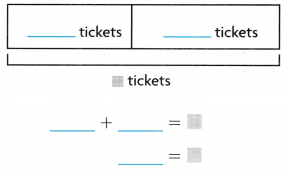Explanation:
261 tickets sold by two grades

Problem Solving

Use the table for 2-5. You may use models, number lines, and equations to represent and solve.Question 2.
How many picture frames were sold at the Craft Fair?
Answer: 180 picture frames were sold at the Craft Fair
Explanation:
180 picture frames were sold at the Craft Fair, Since 108 +72 = 180

Question 3.
How many candles were sold at the Craft Fair?
Answer: 196 candles were sold at the Craft Fair
Explanation:
196 candles were sold at the Craft Fair, Since 104+92= 196

Question 4.
How many flower baskets and candles combined were sold on Sunday?
Explanation:
188 flower baskets and candles combined were sold on Sunday, Since 113 +75 = 188

Question 5.
If another 40 pencil holders were sold after the fair ended, how many pencil holders were sold in all?
Answer: If another 40 pencil holders were sold after the fair ended, 199 pencil holders were sold in all
Explanation:
If another 40 pencil holders were sold after the fair ended, 199 pencil holders were sold in all,
Since 84+75+40 = 199

Lesson Check

Fill in the bubble completely to show your answer. You may use models, number lines, and equations to represent and solve.

Question 6.
Beth reads 45 pages of a book in one week, and 66 pages the following week. How many pages does Beth read in all?
(A) 100
(B) 101
(C) 90
(D) 111
Explanation:
Beth reads 45 pages of a book in one week, and 66 pages the following week. 111 pages Beth read in all.
Since 45 +66= 111

Question 7.
A museum has 165 oil paintings and 128 watercolor paintings. How many paintings does the museum have in all?
(A) 383
(B) 283
(C) 293
(D) 193
Explanation:
A museum has 165 oil paintings and 128 watercolor paintings. Total 293 paintings in the museum have in all.
Since 165 +128=293

Question 8.
Pieto collects 89 bottles for recycling day. Ming collects 68 bottles. What is the total number of bottles that the children collect?
(A) 147
(B) 117
(C) 157
(D) 114
Explanation:
Pieto collects 89 bottles for recycling day. Ming collects 68 bottles. So  the total 157 number of bottles that the children collected. Since 89+68 =157

Question 9.
Multi-Step Mrs. Stein’s classroom has 135 fiction books and 98 nonfiction books. Mr. Walter’s classroom has 29 fewer books. How many books are in Mr. Walter’s classroom?
(A) 194
(B) 204
(C) 233
(D) 262
Explanation:
Mrs. Stein’s classroom has 135 fiction books and 98 nonfiction books. Mr. Walter’s classroom has 29 fewer books. So 204 books are in Mr. Walter’s classroom. Since 135 + 98-29 =204

Question 10.
Multi-Step The table shows the number of people that attended the School Fair. On which day did the most people attend the fair?(A) Sunday
(B) Friday
(C) Saturday
(D) Thursday